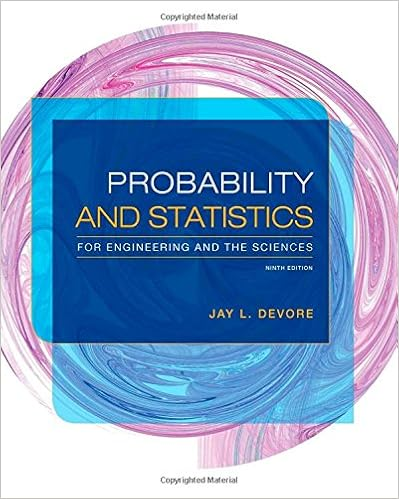# SimulationNotesWeek1.pdf - 1 Week 1 1.1 Agenda First Class...

• Notes
• 6
• 100% (1) 1 out of 1 people found this document helpful

This preview shows page 1 - 3 out of 6 pages.

##### We have textbook solutions for you!
The document you are viewing contains questions related to this textbook.The document you are viewing contains questions related to this textbook.
Chapter 4 / Exercise 3
Probability and Statistics for Engineering and the Sciences
DevoreExpert Verified
1Week 11.1AgendaFirst Class:1. Textbooks:ASM textbook: Applied Simulation Modeling, by Seila, Ceric and Tadikamalla, DuxburyPress, 2003Excel 2010: Data Analysis and Business Modeling, Winston, Microsoft Press, 2011, or lateredition. (For different editions of the Excel book, the chapter numbers may differ, but thechapter name and contents are the same)2. In-class exercise: Dart Game. Please bring your laptop with Excel to every class. We use PC.For Mac, here are the keyboard shortcuts: -shortcuts-in-Excel-2016-for-Mac-acf5419e-1f87-444d-962f-4e951a658ccd3. Reading: Syllabus and the tentative schedule.4. HW1, 1 problem, due on iLearn at noon on 4/11.Estimate pi (ASM textbook chapters 2.1, 2.4):(a). For sample size (or replications) n=100, find 95% confidence interval and the mean forpi estimation;(b). Repeat (a) for sample size n= 500, 1000, 5000;(c). Plot mean, and confidence interval against n. Is the width of the interval increasing ordecreasing as n increases?All homework should be submitted to iLearn at the stated time.You must submit yourown work with appropriate acknowledgement.Save your solutions in a single file, e.g.,HW1.YourName.xls.5. Please sign-up your team online. The team size is three or less. Deadline is 4/11.Second Class:1. Reading before the class:Required: Excel book, ch. 69 (introduction to simulation), ch. 62 (random variables), ch. 63(Binomial), ch. 65 (Normal); Optional: Excel book, ch. 2 (range names), ch. 12 (IF state-ments);2. In-class game: random variable generation, dinner bet.Random variables: Bernoulli(p=0.4), Binomial(n=10, p=0.4), Continuous uniform (a=1, b=12),discrete uniform (a=1, b=12), Triangular (a=1, c=7, b=12), Normal(mu=7, sigma=3), exponen-tial (mu=7), Poisson(mu=7).For each of the following random variables (populations),a) plot its probability distribution;1
##### We have textbook solutions for you!
The document you are viewing contains questions related to this textbook.The document you are viewing contains questions related to this textbook.
Chapter 4 / Exercise 3
Probability and Statistics for Engineering and the Sciences
DevoreExpert Verified
b) generate a sample with size n=100 (number of observations) from the population (randomvariable);c) Compute the mean and its 95 percent confidence interval;d) Plot the histogram (the relative frequency) of the sample, and compare it with the proba-bility distribution of the population (random variable) in a);1.2IntroductionSimulation is a way of thinking. It tells stories in a logical way. Just as every story has actors, plots,and contexts, so does simulation. In a story, we want to know how the actors interact, under whatcontext, and what are the outcome of their interactions. In simulation,random variablesare ouractors, and we seek to understand how and why their interplay leads to certain outcomes.For each simulation, we must first specify the relevant random variables. Like actors, they eachhave names and behaviors (personalities, characters). For example, Bernoulli, Binomial, and Nor-mal are the names of typical random variables.Their behaviors are uniquely defined by theirdistribution functions—probability distribution function (PDF)f, or cumulative distribution func-tion (CDF)F. Depending on the problem, either one can be pleasant to work with. Their relationisF(x) =x−∞f(t)dtfor continuous case, andF(x) =tixf(ti).
•••